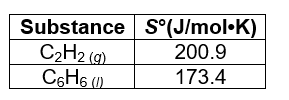# Problem: Calculate the standard entropy, ΔS°rxn, of the following reaction at 25.0°C using the data in this table. The standard enthalpy of the reaction, ΔH°rxn, is -633.1 kJ•mol-1. 3C2H2(g) → C6H6(I) ΔS°rxn =Then, calculate the standard Gibbs free energy of the reaction, ΔG°rxn.ΔG°rxn = Finally, determine which direction the reaction is spontaneous as written at 25.0°C and standard pressure. a. forward b. reverse c. both d. neither

###### FREE Expert Solution

For this problem, we need to solve for ΔS°rxn, ΔG°rxn, and determine which direction the reaction is spontaneous.

For the reaction at T = 25.0°C:

3C2H2(g) → C6H6(I)

The ΔH°rxn = -633.1 kJ•mol-1 (or kJ/mol)

Recall that the equation for the standard entropy, ΔS°rxn is:

The standard free energy change of a reaction (ΔG˚rxn) is given by the following equation:

From the given table, we can find the ΔS˚ of each reactant and product and use these values to calculate ΔS˚rxn95% (230 ratings)###### Problem Details

Calculate the standard entropy, ΔS°rxn, of the following reaction at 25.0°C using the data in this table. The standard enthalpy of the reaction, ΔH°rxn, is -633.1 kJ•mol-1

3C2H2(g) → C6H6(I)

ΔS°rxn =

Then, calculate the standard Gibbs free energy of the reaction, ΔG°rxn.

ΔG°rxn =

Finally, determine which direction the reaction is spontaneous as written at 25.0°C and standard pressure.

a. forward

b. reverse

c. both

d. neither